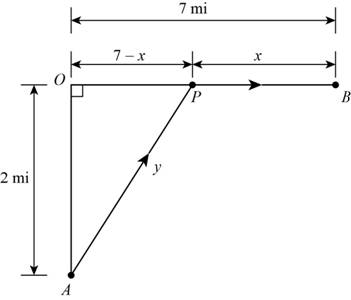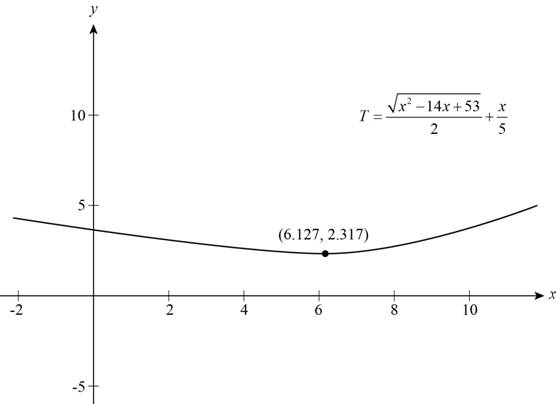# The function that models the time needed by the man for the trip.### Precalculus: Mathematics for Calcu...

6th Edition
Stewart + 5 others
Publisher: Cengage Learning
ISBN: 9780840068071### Precalculus: Mathematics for Calcu...

6th Edition
Stewart + 5 others
Publisher: Cengage Learning
ISBN: 9780840068071

#### Solutions

Chapter 2, Problem 30P

(a)

To determine

## To find: The function that models the time needed by the man for the trip.

Expert Solution

The function that models the time taken by the man is T=x214x+532+x5 .

### Explanation of Solution

Given:

Width of river is 2mi , speed of boat (s) is 2mi/hr and the speed (sm) at which the man walk is 5mi/hr .

Calculation:

Draw the figure according to given situation in the question as shown below.Figure (1)

In the Figure (1) A is the starting point of the trip and B is the end point of the trip.

The man reaches at point P from A in boat and walks the distance PB as shown in the Figure (1).

Width of river (OA) is 2mi and distance between point O and B is 7mi .

Total time of the trip is sum of time taken to cross the river and time taken to walk the remaining distance.

Let time taken to cross the river be t1 and time taken to walk is t2 .

Total time (T) is,

T=t1+t2

The formula to calculate t1 is,

t1=DistancetravelledbytheboatSpeedofboat=ys (1)

Distance travelled by the boat is y .

Apply Pythagorean Theorem in triangle OAP shown in Figure (1).

(AP)2=(OA)2+(OP)2

Substitute y for AP , 2 for OA and 7x for OP in above equation.

(y)2=(2)2+(7x)2y2=4+4914x+x2[(ab)2=a22ab+b2]=5314x+x2

Take square root of above equation.

y=x214x+53

Substitute x214x+53 for y and 2 for s in equation (1) and calculate t1 .

t1=x214x+532

The formula to calculate t2 is,

t2=WalkingdistanceSpeedofman=xsm

Substitute 5 for sm in above equation

t2=x5

Summarize all the information in the table as shown below.

 In Words In Algebra Total time for the trip T Time taken to cross the river x2−14x+532 Time taken in walking x5

Use the information in the table and model the function.

T=t1+t2T=x214x+532+x5

Thus, the function that models the time taken by the man is T=x214x+532+x5 .

(b)

To determine

### To find: The point at which the man should reach to minimize the time of trip.

Expert Solution

The distance is 6.127mi from point B to minimize the time of trip.

### Explanation of Solution

The function that models the time as calculated in part(a) is T=x214x+532+x5

Sketch the graph of the function as shown below.Figure (2)

Observe from the graph that function attains a minimum value when x is 6.127 .

Thus, man should land at distance of 6.127mi from point B .

### Have a homework question?

Subscribe to bartleby learn! Ask subject matter experts 30 homework questions each month. Plus, you’ll have access to millions of step-by-step textbook answers!